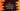# C++ program to format a phone number## C++ program for phone number formatting:

In this post, we will learn how to do phone number formatting in C++. In this post, we will use phone number format for US. USA phone numbers are divided in area code, exchange code and line number. It looks like +1-650-511-0215, where 650 is the area code, 511 is the exchange code and 0215 is the line number.

Our program will take the inputs from the user and print it. It will use one structure to hold the values of the phone numbers. This program is for US phones but you can modify it to other country’s phone numbers as well.

### C++ program:

``````#include <iostream>

using namespace std;

struct PhoneNumber
{
int areaCode, exchangeCode, lineNumber;
};

int main()
{
PhoneNumber number;
cout << "Enter the area code, exchange code and line number separated by space :" << endl;
cin >> number.areaCode >> number.exchangeCode >> number.lineNumber;
cout << "Formatted phone number : "
<< "+1-" << number.areaCode << "-" << number.exchangeCode << "-" << number.lineNumber<<endl;
}``````

Here,

• PhoneNumber is the structure to store the area code, exchange code and line number for a phone.
• The program asks the user to enter the area code, exchange code and line number separated each value by a space. It reads the values and stores in the PhoneNumber structure variable number.
• The last line prints the formatted phone number by joining the values of number variable.

### Sample output:

This program will print output as like below:

``````Enter the area code, exchange code and line number separated by space :
650 422 1234
Formatted phone number : +1-650-422-1234``````